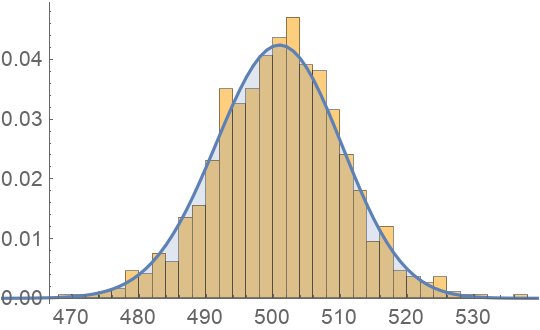#Function Repository Resource:

# RunCountRandomnessTest

Conduct a runs up–based randomness test on a sequence of random reals between 0 and 1

Contributed by: Emmy/Noah Blumenthal
noahb320@gmail.com
emmyb320@bu.edu
 ResourceFunction["RunCountRandomnessTest"][sequence] counts the number of increasing runs to assess randomness of sequence and returns an associated p value. RunLengthRandomnessTest[sequence,"property"] counts the number of increasing runs to assess randomness and returns the specified property.

## Details and Options

Properties include:
 "TestStatistic" returns the test statistic "PValue" returns the p value associated with the test
The test statistic is generated by creating a chi square–like statistic that measures the difference between the counts of runs up in a sequence and the expected mean counts of runs up in the sequence.
The test only works for sequences of random reals between 0 and 1.
RunCountRandomnessTest results are valid only for sequence lengths greater than 600.
The RunCountRandomnessTest function performs a two-tailed test on the test statistic.

## Examples

### Basic Examples

Generate a sequence of random integers and apply a run count–based test:

 In:=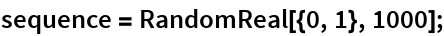Visualize the sequence:

 In:=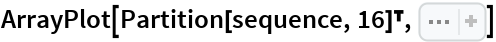Out=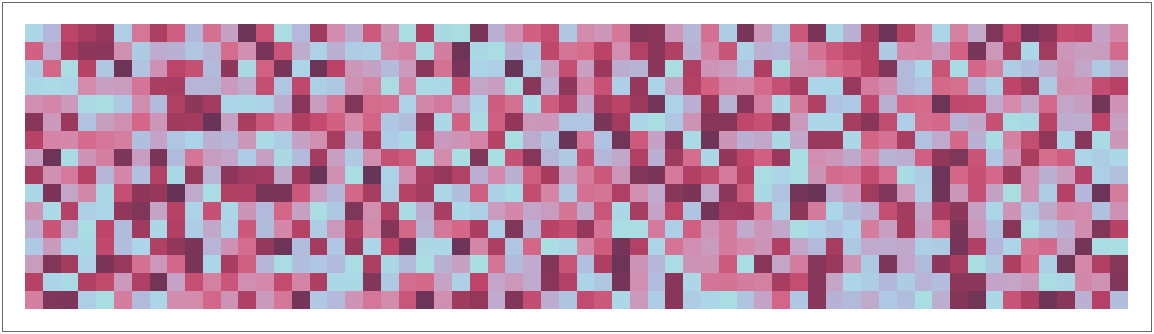In:=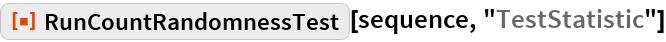Out=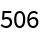In:=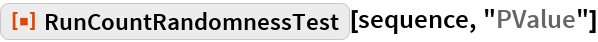Out=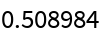### Scope

Attempt to reject a nonrandom sequence:

 In:=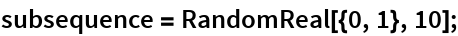In:=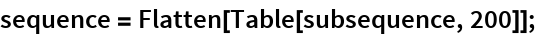In:=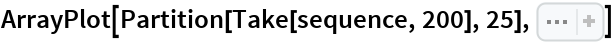Out=In:=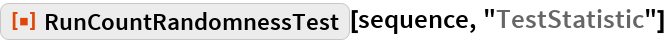Out=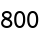In:=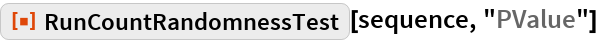Out=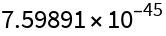### Possible Issues

This test is not very powerful and fails to reject nonrandom sequences that repeat over longer intervals on occasion; ideally, it should be used in conjunction with another test:

 In:=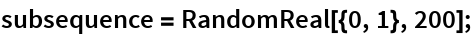In:=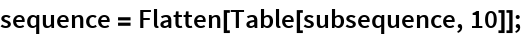In:=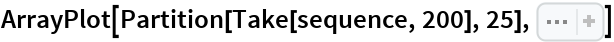Out=In:=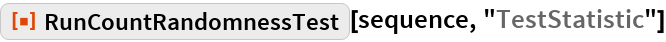Out=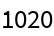In:=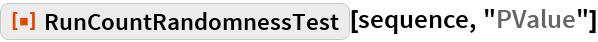Out=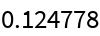### Neat Examples

Visualize the sampling distribution of the test statistic:

 In:=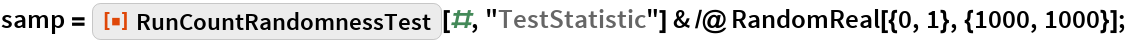In:=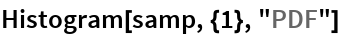Out=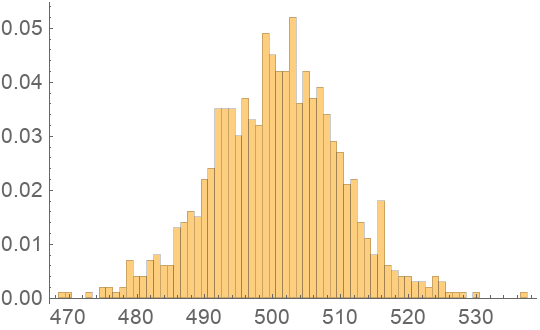In:=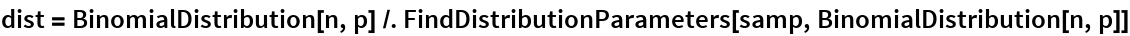Out=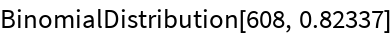In:=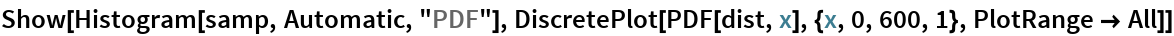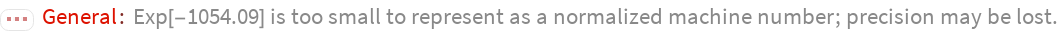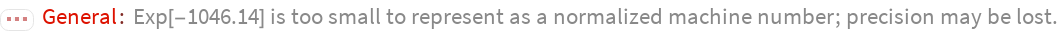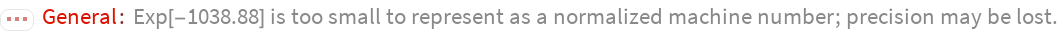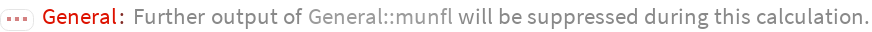Out=